## LetsPlayMaths.Com

WELCOME TO THE WORLD OF MATHEMATICS

# Class 4 Division

Division of 6-Digit Numbers by One Digit Number

Division of 6-Digit Numbers by Two Digit Number

Division of 6-Digit Numbers by Multiples of 10

Story Time Division

Division Test

Division Worksheet

## Division of 6-Digit Numbers by One Digit Number

In previous class, we have learnt division of 4/5 digit numbers by one-digit numbers. We will use same
method for dividing six-digit numbers by one-digit numbers. Few examples are given below.

Example 1. Divide 275625 by 5.
Solution.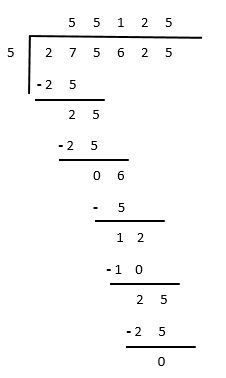So, the answer is 55125.

Example 2. Divide 730201 by 9.
Solution.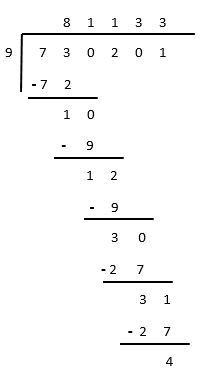So, the quotient is 81133 and remainder is 4.

## Division of 6-Digit Numbers by Two Digit Number

All the rules that we have learnt earlier will be used in this kind of division. Here, we will have 2 digits
divisor. When we divide a number by a 2-digit number whose table we do not know, we must multiply to get
the answer. Let’s see some examples.

Example 1. Divide 488244 by 12.
Solution.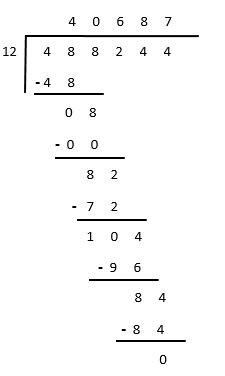So, the quotient is 40687.

Example 2. Divide 968727 by 25.
Solution.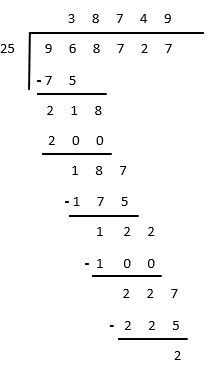So, the quotient is 38749 and remainder is 2.

## Division of 6-Digit Numbers by Multiples of 10

Now let us learn to divide 6-digit numbers by 2-digit numbers ending with zero. Few examples are given below.

Example 1. Divide 265750 by 50.
Solution.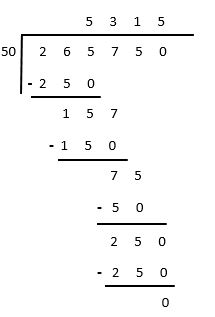So, the quotient is 5315.

Example 2. Divide 336735 by 30.
Solution.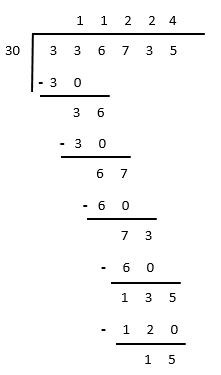So, the quotient is 11224 and remainder is 15.

## Story Time Division

Story time divisions are solved in the similar manner as studied in previous classes. Here, we will be using
larger numbers. Few examples are given below.

Example 1. 9 motorcycle costs ₹ 729576. What is the cost of one motorcycle?
Solution.
Cost of 9 motorcycles = ₹ 729576
Cost of 1 motorcycle = ₹ 729576 ÷ 9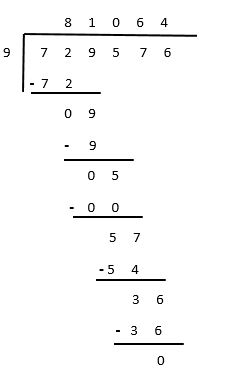So, the cost of one motorcycle is ₹ 81064.

Example 2. 507680 rupees to be distributed equally among 32 families of a village. How much money each family
Total money to be distributed among 32 families = ₹ 507680
Each family will receive = ₹ 507680 ÷ 32Thus, each family will receive ₹ 15064.

## Division Test

Division Test - 1

Division Test - 2

## Class-4 Division Worksheet

Division Worksheet - 1

Division Worksheet - 2

Division Worksheet - 3